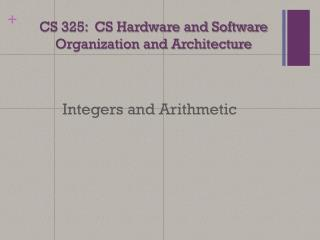Download PresentationCS 325: CS Hardware and Software Organization and Architecture

# CS 325: CS Hardware and Software Organization and Architecture - PowerPoint PPT PresentationDownload Presentation## CS 325: CS Hardware and Software Organization and Architecture

- - - - - - - - - - - - - - - - - - - - - - - - - - - E N D - - - - - - - - - - - - - - - - - - - - - - - - - - -
##### Presentation Transcript

1. CS 325: CS Hardware and SoftwareOrganization and Architecture Integers and Arithmetic

2. Outline • Number Representation • Decimal • Binary • Hexadecimal • Decimal vs. Hexadecimal vs. Binary • Number Conversions • Dec  Bin, Dec  Hex • Bin  Dec, Bin  Hex • Hex  Dec, Hex  Bin

3. Decimal Numbers: Base 10 • Digits: 0, 1, 2, 3, 4, 5, 6, 7, 8, 9 • Example: 4923 = (4x103) + (9x102) + (2x101) + (3x100)

4. Number Base: • Number with base x  x digits: • Base 10 (Decimal): 0, 1, 2, 3, 4 ,5 ,6 ,7 , 8, 9 • Base 2 (Binary): 0, 1 • Number representation: • d31d30d29….d2d1d0 is a 32 digit number • 4326210 is a 5 digit base 10 (Dec) number • 101011010112 is a 11 digit base 2 (Bin) number

5. Binary Numbers: Base 2 • Digits: 0, 1 • Example: 101011= (1x25) + (0x24) + (1x23) + (0x22) + (1x21) + (1x20) = 4310 • What about a base that converts to binary easily?

6. Hexadecimal Numbers: Base 16 • Digits: 0, 1, 2, 3, 4, 5, 6, 7, 8, 9, A, B, C, D, E, F • Decimal digits + A – F • Example: 12E = (1x162) + (2x161) + (Ex160) = 30210

7. Decimal vs. Hexadecimal vs. Binary

8. Number Conversion: Dec  Bin • Converting from base 10 to base 2: Continue dividing decimal number by 2 and keep the remainder • Example: 3510 1000112

9. Number Conversion: Dec  Bin • Example: • Convert 42310to Bin 1101001112

10. Number Conversion: Dec  Hex • Converting from base 10 to base 16: • Example: 3510 2316

11. Number Conversion: Dec  Hex • Example: • Convert 21010to Hex D216

12. Number Conversion: Bin  Dec • Converting from base 2 to base 10: • Example: 110102 (1x24) + (1x23) + (0x22) + (1x21) + (0x20) 16 + 8 + 0 + 2 + 0 = 2610

13. Number Conversion: Bin Dec • Example: • Convert 101011102to Dec 17410

14. Number Conversion: Bin  Hex • Converting from base 2 to base 16: • Example: 110101102 • 1 Hex digit represents 16 Decimal values • 4 Binary digits represent 16 Decimal values • 1 Hex digit replaces 4 Binary digits D616

15. Number Conversion: Bin Hex • Example: • Convert 110011112to Hex CF16

16. Number Conversion: Hex  Dec • Converting from base 16 to base 10: • Example: 8E316 (8x162) + (Ex161) + (3x160) 2048 + 224 + 3 = 227510

17. Number Conversion: Hex  Dec • Example: • Convert 63F16to Dec 159910

18. Number Conversion: Hex  Bin • Converting from base 16 to base 2: • Example: 9A2E16 10011010001011102

19. Number Conversion: Hex  Bin • Example: • Convert 26FA16to Bin 100110111110102

20. What to do with representations of numbers? • add, subtract, multiply, divide, compare • Example: 8 + 6 = 14 1 0 0 0 +0 1 1 0 1 1 1 0 Simple enough to add in binary that we can build circuits to do it.

21. Which base do we use? • Decimal: Great for human, especially when doing arithmetic • Hex: Easier for humans to read than long strings of binary numbers. Easy to convert to binary, each hex decimal = 4 binary bits. • Binary: used by all computers. Bin represents an abstraction…but and abstraction of what?

22. The Transistor • A controlled switch. • Collector – positive lead • Emitter – negative lead • Base – control lead • A binary “1” represents an active transistor.

23. The Transistor

24. Limits of Computer Numbers • Bits can represent anything • Characters • ‘a’, ‘F’ • 7 bit ASCII, 8 bit Extended ASCII • Logical Values • 0  False, 1 True • Colors? • Locations/addresses? Commands? • But N bits  only 2N things